# Sample function

(diff) ← Older revision | Latest revision (diff) | Newer revision → (diff)

sample path

A function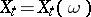of an argumentwhich unambiguously corresponds to each observation of a random process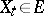,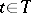, where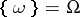is a set of elementary events. The terms "realization of a random processrealization" and "trajectory of a random processtrajectory" , which are equivalent to "sample function" and "sample path" , are also frequently employed. A random process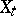is characterized by a probability measure in the space of the sample function. In studying the local properties of the sample function(where, and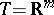is the Euclidean space of dimension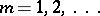) it is assumed thatis a separable random process or that an equivalent random process with given local properties of the sample function can be found. The local properties of the sample functions of Gaussian processes (cf. Gaussian process) have been most extensively studied.

For Gaussian random processes (fields)the following holds: Almost all sample functionsare either continuous or unbounded over some interval. For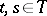a "distance" is defined by,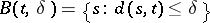is a "ball" , andis the minimum number of such "balls" which cover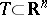, further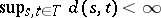. A necessary and sufficient condition for the continuity of the sample function of a homogeneous Gaussian process has the form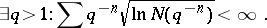If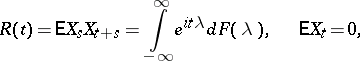is concave in some neighbourhood of the point, then for the sample functionto be continuous it is necessary and sufficient that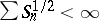, where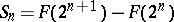. Ifis concave in a neighbourhood ofand if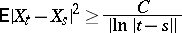for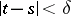, almost all sample functions of the Gaussian random processare unbounded. Ifalmost all sample functions of the Gaussian random process (field)are continuous. For the sample function of a Gaussian random process to be continuous it is necessary and sufficient that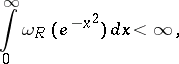where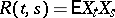,Here, the supremum is taken over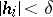,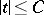,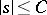. The sample function,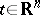, is in the class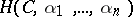if for all sufficiently small,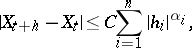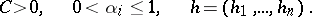If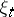is a Gaussian random field on the unit cube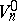insuch that for sufficiently smalland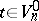,then, with probability one, uniformly in,for anyand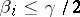.

A non-decreasing continuous function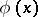,, is called an upper function if for almost allthere exists an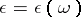such that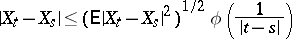for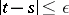;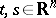;. Ifis a Gaussian random field withthenis an upper function if and only if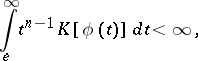where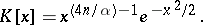For almost all sample functions of a Gaussian random process to be analytic in a neighbourhood of a point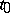it is necessary and sufficient that the covariance function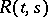be analytic inandin a neighbourhood,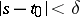,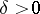.

How to Cite This Entry:
Sample function. Encyclopedia of Mathematics. URL: http://encyclopediaofmath.org/index.php?title=Sample_function&oldid=13989
This article was adapted from an original article by Yu.K. Belyaev (originator), which appeared in Encyclopedia of Mathematics - ISBN 1402006098. See original article